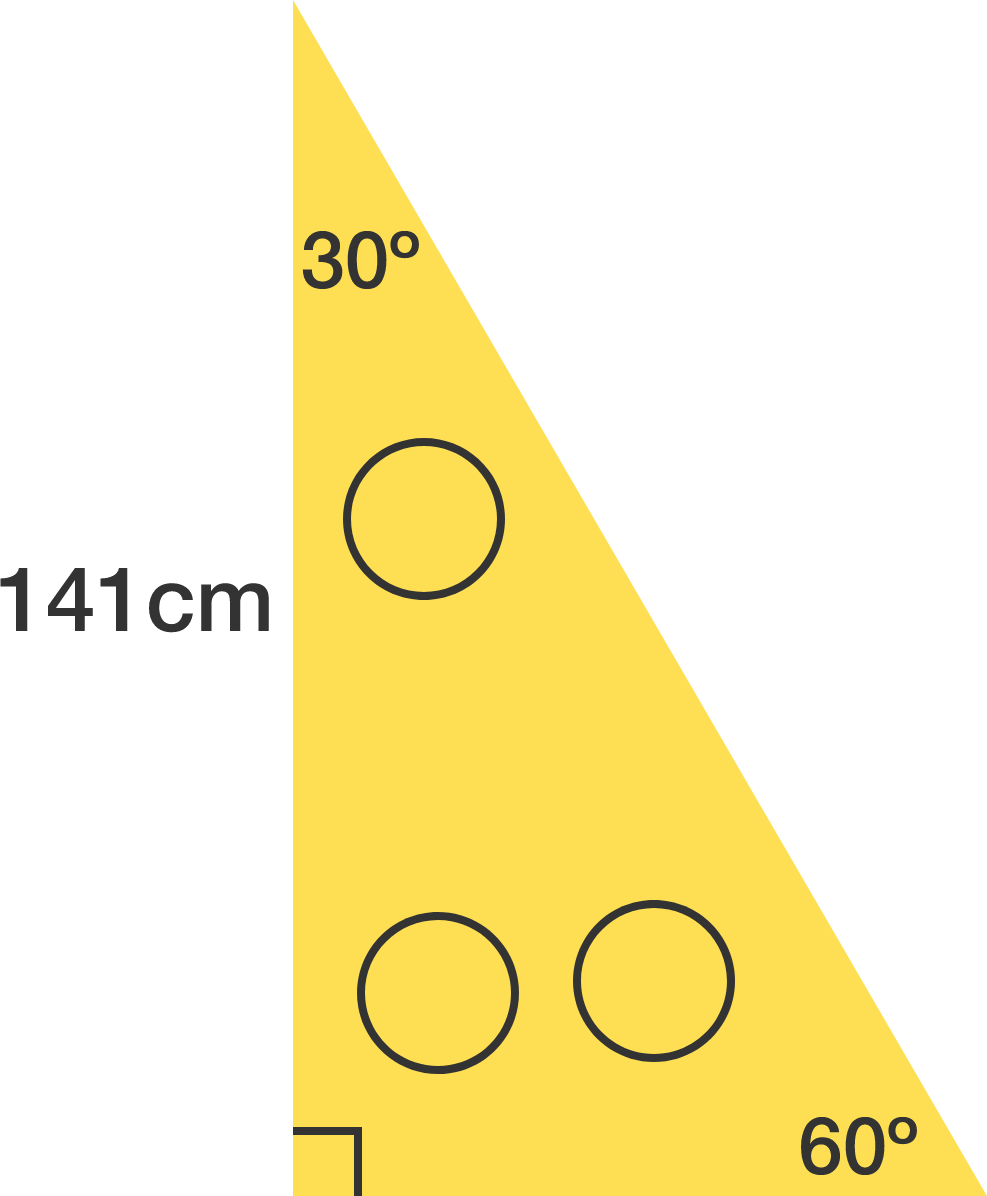# Packing circles in a triangle

Geometry Level 3

Three identical circles are cut out from a 30$^\circ$-60$^\circ$-90$^\circ$ right triangle with the second largest side length 141 centimeters.

To three decimal places, what is the largest possible radius of these circles in centimeters?×